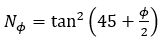# Weak Rock

The following properties are required or available for weak rock layers:

• Unconfined Compressive Strength (quc)
• Reduction Factors (%) – percent decrease in computed unit resistance for layer
• Skin Resistance Loss
• End Bearing Loss
• Unit Resistance Limits – maximum value of unit resistance
• Skin Friction Limit
• End Bearing Limit

Note that the skin friction resistance is capped by 5% of the entered Concrete Cylinder Strength in the pile properties.

The following parameters are required specifically for each method as described below. For more information see Bored Capacity Theory Manual

For weak rock models, you can combine any skin resistance model with any end-bearing model. The following parameters are required for each method.

## Skin Resistance Methods:

### WILLIAM AND PELLS

• Average RQD - average rock quality designation

The ultimate unit skin (frictional) resistance (fs,ult) is computed as follows:

fs,ult =α β quc

Where:

α = 0.5 quc-0.5, adhesion factor of the intact rock based on the recommended graph below (quc in MPa units)

β = reduction factor that is related to the mass continuity factor j as shown below

j = mass continuity factor obtained from RQD value as shown below

See the theory manual for more details.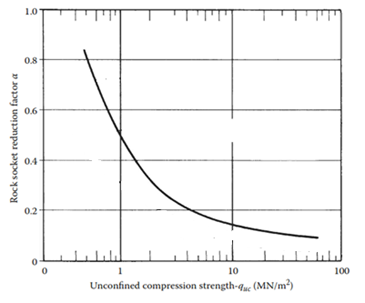The adhesion factor of intact weak rocks (mudstone, shale, sandstone, etc.) from Williams and Pells 1981.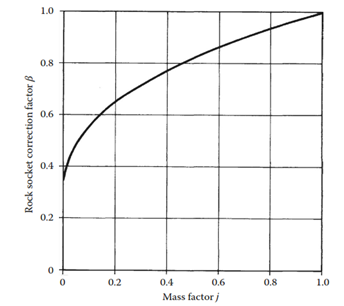The correction factor β in Williams and Pells method.

Mass factor j

 0-25 > 15 0.2 25-50 15-8 0.2 50-75 8-5 0.2-0.5 75-90 5-1 0.5-0.8 90-100 1 0.8-1

Mass factor j values corresponding to RQD

### KULHAWY AND PHOON

• Value of Chi (χ)

The ultimate unit skin (frictional) resistance (fs,ult ) is computed as follows: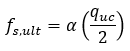Where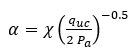Pa = atmospheric pressure to normalize values that is taken as 100 kPa.

## Tip Resistance Methods:

### USER-DEFINED B

• b parameter – see theory manual for more details

The ultimate unit end (base) resistance (qb,ult) is computed as follows: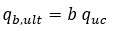### ZHANG AND EINSTEIN

The ultimate unit end (base) resistance (qb,ult) is computed as follows: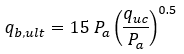Pa = atmospheric pressure to normalize values that are taken as 100 kPa.

### TOMLINSON N PHI

• Internal friction angle (ϕ)

The ultimate unit end (base) resistance (qb,ult) is computed as follows: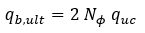Where: